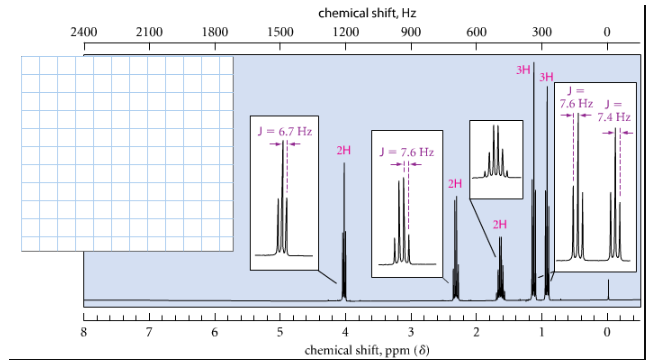# Problem: Provide a structure for the following compound:C6H12O2:IR: 1743 cm-1; 1H NMR spectrum:

###### FREE Expert Solution

Index of hydrogen deficiency (IHD)  # of missing H's in structure:

$\overline{){\mathbf{IHD}}{\mathbf{=}}\frac{\mathbf{\left[}\mathbf{2}\mathbf{n}\mathbf{+}\mathbf{2}\mathbf{\right]}\mathbf{-}\mathbf{H}}{\mathbf{2}}}$

where n = number of carbons and H is:

+1 for hydrogen (H) or halogens (X)

0 for oxygen (O)

–1 for nitrogen (N)

Given:C6H12O2

$\mathbf{IHD}\mathbf{=}\frac{\mathbf{\left[}\mathbf{2}\left(6\right)\mathbf{+}\mathbf{2}\mathbf{\right]}\mathbf{-}\mathbf{12}}{\mathbf{2}}$

IHD = 1 → ring or double bond

IR spectra: 1735 cm-1 ester (RCOOR: 1735-1750 cm-1 double bond (C=O)

96% (78 ratings)###### Problem Details

Provide a structure for the following compound:

C6H12O2:

IR: 1743 cm-1

1H NMR spectrum: